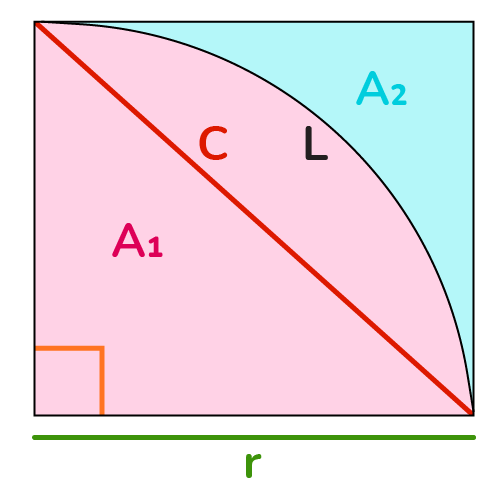# Quarter Circle Calculator

Created by Komal Rafay
Reviewed by Anna Szczepanek, PhD
Last updated: Feb 02, 2023

Our quarter circle calculator is a solution to so many problems and concerns you have for the quarter of a circle. The quarter of a circle is a simple shape yet has many parameters: we will try to cover them all: from the area and perimeter to the chord of a quarter circle.

## Quarter circle calculator

As much as we love to eat cakes, it's rarely a chance that we ever get a whole quarter, and before you know it, we are craving for more. But our tool is your friend, and it will not let you crave anything.
So, you want to determine all you can about the quarter circle. The only thing our tools ask of you is to input the radius of the quarter circle. And the list of results is ready.

For instance, you input the radius as 13 cm. The results are:

1. Chord = $18.38 \text{ cm}$
2. Quarter arc = $20.42\text{ cm}$
3. Perimeter = $46.42\text{ cm}$
4. Quarter area = $132.73\text{ cm}^2$
5. External area= $36.27\text{ cm}^2$

## Features of a quarter of a circle

The features of the quarter circle are the parameters of a quarter that are provided in our tool. Let's dive in and look at them individually and also their formulas.

• Chord of a quarter circle
The chord of the circle is a line segment joining the two radii. It makes it seem like a right-angled triangle inside the quarter. The formula to determine the chord is:

$c = r \times \sqrt{2}$

• Arc of a quarter circle
The arc of a quarter circle is the arc that would be the part of the circumference of the circle. It is the curved line joining the two radii. The formula to calculate the arc length is:

$L = \frac{1}{2} \times \pi \times r$

• Perimeter of a quarter circle
The perimeter of a quarter circle is the outer boundary of the quarter, it includes the 2 radii and the arc. The formula you may use to find out the perimeter is:
$P = L + (2 \times r)$

• Area of a quarter circle
The area of the circle is, as the name indicates, the space covered by the quarter circle. The formula to figure out the area is:
$A_1 = \frac{1}{4} \times \pi \times {r^2}$

• External area of a quarter circle
The external area is the area outside the quarter itself. The formula for the external area is:
$A_2 = r^2 - A_1$

where:

• $r$ - Radius of the quarter circle;
• $c$ - Chord of the quarter circle;
• $L$ - Arc length of the quarter circle;
• $P$ - Perimeter of the quarter circle;
• $A_1$ - Quarter area; and
• $A_2$ - External area of the quarter circle.

## Area of a quarter circle

Let's take an example of the odd quarter-shaped pot you have on your table, which carries a wonderful baby cactus.
You might wonder how much area it covers among all the clutter.
All you have to do is grab a measuring tape or a ruler from your desk and measure the radius of the pot. Let's say it is $9\text{ cm}$. Put the radius in the formula:

$A_1 = \pi \times \frac{r^2}{4}$

$A_1 = 3.14 \times \frac{9^2}{4}$

$A_1 = 3.14 \times \frac{81}{4}$

$A_1 = 3.14 \times 20.25$

$A_1 = 63.615$

$A_1 = 63.62 \text{ cm}^2$

## The world of a sector of a circle

The sector of a circle is versatile. Could be any segment of a circle to a quarter of a circle. Hence we at Omni have dedicated some tools to the sector of a circle. Check them out!

## FAQ

### How can I find the chord of a quarter circle?

The chord of the quarter circle is a line connecting the two arms. Imagine a right-angled triangle inside the quarter; this triangle's hypotenuse is the chord.
Its formula is:

c = r × √2

where:

• c - The chord of the quarter circle; and
• r - The radius of the quarter circle.

### What is the area of a quarter circle with radius 6 cm?

The area of a quarter circle is 28.27 cm² when the radius measures 6 cm.

Substituting the value for the radius in the formula, we have:

A = π × r²/4

A = 3.14 × (6 × 6 / 4)

A =28.27 cm²

### Can I calculate the quarter of a circle?

The quarter of a circle in itself is a shape. You will have to specify which of its features you want to calculate. There are many parameters of a quarter circle that you can determine using simple formulas. The parameters are:

• Chord;
• Arc length;
• Perimeter;
• Area; and
• External area of a quarter circle if the circle is enclosed in a square.

And for more advanced studying, the centroid of a quarter circle.

Komal Rafay
inChord (c)
in
Quarter arc (L)
in
Perimeter
in
Quarter area (A1)
in²
External area (A2)
in²
People also viewed…

### Chinese remainder theorem

The Chinese remainder theorem calculator is here to find the solution to a set of remainder equations (also called congruences).

### Circumference

Use this free circumference calculator to find the area, circumference and diameter of a circle.

### Greatest common denominator

Quickly calculate the greatest common denominator of a group of numbers with our handy tool!

### Sleep

The sleep calculator can help you determine when you should go to bed to wake up happy and refreshed.